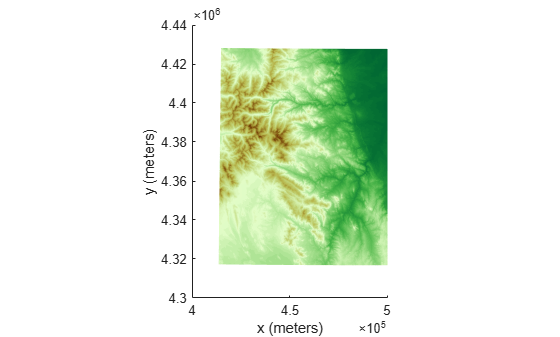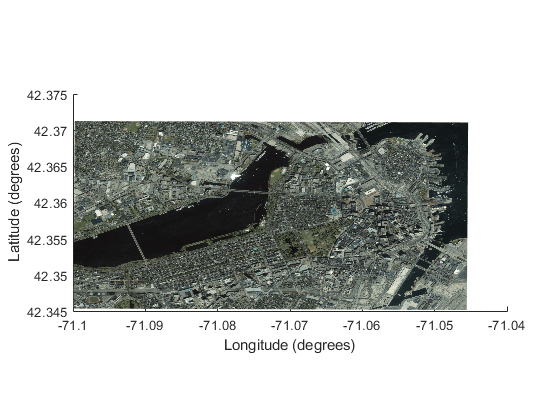## Project and Display Raster Data

To project or unproject regularly-spaced raster data that is associated with a geographic or map reference object, you must first create a coordinate grid that matches the size of the raster. Use different grid creation functions depending on which way you are projecting. When you project latitude-longitude coordinates to x-y coordinates, create a grid using the `meshgrat` function. When you unproject x-y coordinates to latitude-longitude coordinates, create a grid using the `meshgrid` and `intrinsicToWorld` functions.

After transforming the raster data, you can display it on a map using visualization functions such as `mapshow` and `geoshow`. Use `mapshow` for projected x-y coordinates and `geoshow` for unprojected latitude-longitude coordinates.

### Project Raster Data

To project data that is associated with a geographic raster reference object, first create a grid of latitude-longitude coordinates for each point in the raster. Then, project the geographic coordinates to x-y map coordinates.

For example, import elevation raster data as an array and a geographic cells reference object. Get the latitude-longitude coordinates for each point in the raster by using the `meshgrat` function.

```[Z,R] = readgeoraster('n39_w106_3arc_v2.dt1'); [lat,lon] = meshgrat(Z,R);```

Now that you have your grid, select a map projection to use when projecting the coordinates. For this example, create a `projcrs` object for UTM zone 13 in the northern hemisphere. Then, project the latitude-longitude coordinates to x-y coordinates.

```p = projcrs(32613); [x,y] = projfwd(p,lat,lon);```

Display the projected raster as a surface by calling `mapshow` and specifying the x-y coordinates and elevation array. Add axis labels and apply a colormap appropriate for elevation data.

```figure mapshow(x,y,Z,'DisplayType','surface') xlabel('x (meters)') ylabel('y (meters)') demcmap(Z)```If the geographic CRS of the latitude-longitude coordinates does not match the geographic CRS of the projected CRS, then the projected coordinates may be inaccurate. You can find the geographic CRS of a `projcrs` object or a geographic raster reference object by querying their `GeographicCRS` properties.

`p.GeographicCRS.Name`
```ans = "WGS 84" ```
`R.GeographicCRS.Name`
```ans = "WGS 84" ```

The DTED file used in this example is courtesy of the US Geological Survey.

### Unproject Raster Data

To unproject data that is associated with a map raster reference object, first create a grid of x-y coordinates for each point in the raster. Then, unproject the x-y map coordinates to geographic coordinates.

For example, import an image of Boston as an array and a map cells reference object. Get information about the map projection as a `projcrs` object by querying the `ProjectedCRS` property of the reference object.

```[Z,R] = readgeoraster('boston.tif'); p = R.ProjectedCRS;```

Create a coordinate grid by getting the x-y coordinates for each point in the raster. To do this, first create a grid of intrinsic coordinates that matches the size of the raster by using the `meshgrid` function. The intrinsic y-coordinates increase from row to row and the intrinsic x-coordinates increase from column to column. Then, transform the intrinsic coordinates to x-y coordinates by using the `intrinsicToWorld` function.

```s = size(Z); [xIntrinsic,yIntrinsic] = meshgrid(1:s(2),1:s(1)); [x,y] = intrinsicToWorld(R,xIntrinsic,yIntrinsic);```

Unproject the x-y coordinates to latitude-longitude coordinates by using the `projinv` function and specifying the `projcrs` object and coordinate grid.

`[lat,lon] = projinv(p,x,y);`

Display the unprojected image by calling `geoshow` and specifying the latitude-longitude coordinates and image array. By default, `geoshow` displays coordinates using a Plate Carr$\stackrel{´}{\mathrm{e}}$e projection. Then, add axis labels.

```figure geoshow(lat,lon,Z) xlabel('Longitude (degrees)') ylabel('Latitude (degrees)')```## SupportGet trial now